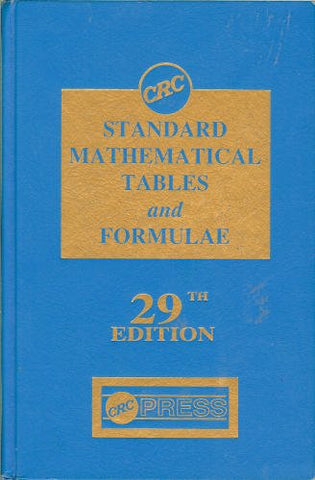# CRC Standard Mathematical Tables and Formulae, 29th Edition (Discrete Mathematics and Its Applications)

• ISBN-13: 9780849306297
• \$13.95

Only 3 left!

Numerical tables of mathematical functions are in continuous demand by students of mathematics and related sciences, instructors, and professional scientists. The 29th Edition of the "CRC Standard Mathematical Tables and Formulae" provides a broad spectrum of mathematical data and essential formulae necessary for today's scientific needs.